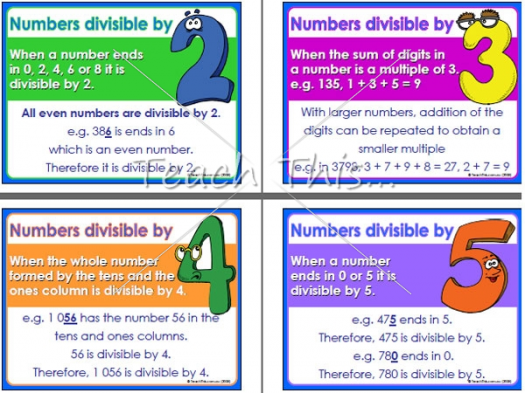# What Do You Know About Dependent And Independent Variables?

10 Questions | Total Attempts: 140SettingsIn mathematical modelling, statistical modelling and experimental sciences, the values of dependent variables depend on the values of independent variables. The dependent variables represent the output or outcome whose variation is being studied. The independent variables represent inputs or causes, i. E. , potential reasons for variation or, in the experimental setting, the variable controlled by the experimenter.

• 1.
Mathematics is usually taught at ....
• A.

School

• B.

Home

• C.

Church

• D.

Mosque

• 2.
Dependent and independent variables is a sub-topic of ....
• A.

Variables

• B.

Proportions

• C.

Deciamals

• D.

Ratios

• 3.
Variables is a topic under ....
• A.

Music

• B.

Maths

• C.

Sociology

• D.

Creative arts

• 4.
Who is not a scientist among them?
• A.

Albert Einstein

• B.

Isaac Newton

• C.

Will Smith

• D.

Bill Nye

• 5.
"Variable" comes from a ..... word
• A.

English

• B.

Latin

• C.

Spanish

• D.

Greek

• 6.
François Viète introduced at the end of ..... the idea of representing known and unknown numbers by letters, nowadays called variables.
• A.

10th century

• B.

14th century

• C.

16th century

• D.

15th century

• 7.
Who invented the convention of representing unknowns in equations by xy, and z, and knowns by ab, and c"?
• A.

François Viète

• B.

René Descartes

• C.

Albert Einstein

• D.

Isaac Newton

• 8.
In ...., René Descartes "invented the convention of representing unknowns in equations by xy, and z, and knowns by ab, and c"
• A.

1637

• B.

1780

• C.

1999

• D.

2001

• 9.
In the second half of the ..... century, it appeared that the foundation of infinitesimal calculus was not formalized enough to deal with apparent paradoxes.
• A.

11th

• B.

12th

• C.

14th

• D.

19th

• 10.
In mathematics, the variables are generally denoted by a single ....
• A.

Letter

• B.

Number

• C.

Symbol

• D.

None

Related TopicsBack to top